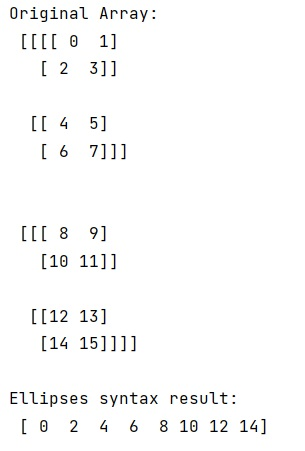# NumPy: Slice of arbitrary dimensions

Learn, how to slice numpy array of arbitrary dimensions in Python?
Submitted by Pranit Sharma, on January 18, 2023

NumPy is an abbreviated form of Numerical Python. It is used for different types of scientific operations in python. Numpy is a vast library in python which is used for almost every kind of scientific or mathematical operation. It is itself an array which is a collection of various methods and functions for processing the arrays.

## Slicing NumPy array of arbitrary dimensions

Suppose that we are given a NumPy array and we need to slice this NumPy array to obtain the ith index in the last dimension.

For this purpose, we will use the Ellipsis technique. The ellipsis is used in NumPy to slice high-dimensional data structures. It is designed to mean at this point, insert as many full slices (:) to extend the multi-dimensional slice to all dimensions.

Suppose that we are given a four-dimensional matrix of order 2 X 2 X 2 x 2. To select all the first elements in the fourth dimension we can use the ellipsis notation. Ellipses are also useful for zero-dimensional data structures.

Let us understand with the help of an example,

## Python code to slice numpy array of arbitrary dimensions

```# Import numpy
import numpy as np

# Creating a numpy array
arr = np.arange(16).reshape(2,2,2,2)

# Display original array
print("Original Array:\n",arr,"\n")

# Using ellipses syntax
res = arr[..., 0].flatten()

# Display result
print("Ellipses syntax result:\n",res,"\n")
```

Output:What's New (MCQs)

Top Interview Coding Problems/Challenges!

IncludeHelp's Blogs

Languages: » C » C++ » C++ STL » Java » Data Structure » C#.Net » Android » Kotlin » SQL
Web Technologies: » PHP » Python » JavaScript » CSS » Ajax » Node.js » Web programming/HTML
Solved programs: » C » C++ » DS » Java » C#
Aptitude que. & ans.: » C » C++ » Java » DBMS
Interview que. & ans.: » C » Embedded C » Java » SEO » HR
CS Subjects: » CS Basics » O.S. » Networks » DBMS » Embedded Systems » Cloud Computing
» Machine learning » CS Organizations » Linux » DOS
More: » Articles » Puzzles » News/Updates